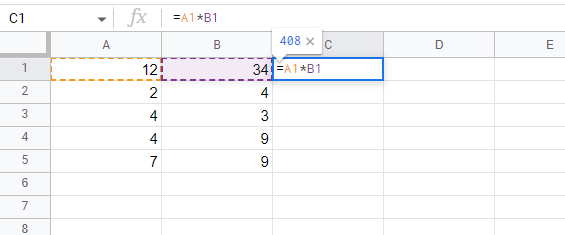# How to create a simple formula in Google Sheets?

Published June 16, 2022

If you have a lot of numerical data you wish to calculate, Google Sheet is one of the best applications you can use. You can create and edit simple formulas in Google Sheets for adding, subtracting, multiplying, and dividing. As you read this article, you will learn how to create and edit formulas that will enable you to perform simple mathematical operations.

## How to create and edit simple formulas

When creating an arithmetic formula, be sure to use arithmetic operators such as subtract, add, divide, and multiply. In addition to this, you will also refer to a cell's address, known as a cell reference, to refer to a particular number within that cell. By using cell references, you can ensure that the values in your formulas are accurate.

## The Mathematical operators to use

Formulas in Google Sheets include the obvious mathematical operators like (+) plus sign for addition, (/) slash for division, (-) minus sign for subtraction, (*) caret for exponents, and (*) star for multiplication.

To create a formula, you need to start with an equals sign (=). As an example, to add two numbers in cells A1 and B1, you would simply create the formula = A1 + A2

## How to Create Formulas

We will now create simple formulas using the mathematical operators and cell references we have discussed above. Now let's take a look at how to set them up.

Step 1: First, select the cells that you would like the calculated value displayed. The calculated answer or solution will be displayed.

Step 2: Now, we will use the cell references to represent the numerical values in the cells we would like to use in the formula. As we said, simple formulas usually comprise one mathematical operator. In this case, we are going to multiply two numbers, the numbers in  column A and  the numbers in column B

Step 3: Now, in C1, enter the simple formula to multiply the two numbers. To do so,  enter the equals sign (=) followed by the cell references of the two cells. In this case, we are going to enter the formula =A1*A1Step 4: Now press enter on your keyboard to generate  the answerStep 5: Now, click on the fill handle and drag down the column C to copy and paste the formula to the rest of the cells (C2 to C5).Once you release your mouse, the answers to the multiplication of the two numbers will be pasted on the selected cells.Conclusion

By default, Google Sheets will not display the formula we have created, and this makes it easier to make errors. To view these formulas, double-click on the cells with the formula, and you will see the formulas. From there, you can verify whether the formulas are correct

 Article Contributed By :48 Views